# Visual Basic Tic-Tac-Toe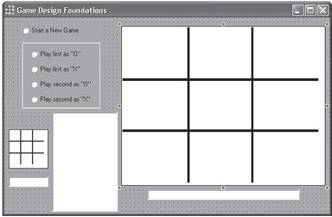Our user interface (above) lets the player start a new game, choosing whether he would like to go first or second as either X or O, and lists the moves (spaces marked). The larger “big” board is where the current game is played and the player marks his desired space. The smaller board displays the computer thinking as calculated by the Min-Max with Alpha-Beta pruning search algorithm.

FORM1

` Private Sub Form_Load() Dim i As Integer Form1.BorderStyle = 0 Text1.Text = " " + "Moves" + " " Frame1.Visible = False InitVariables playerMark = -1 End Sub Private Sub Option1_Click(index As Integer)  Picture1.Enabled = True  Picture1.PaintPicture BigBoard, 0, 0  Picture2.PaintPicture SmBoard, 0, 0  InitVariables  showBigBoard  showSmallBoard  playerMark = (2 * (index Mod 2)) - 1 '-1 is "O", 1 is "X"  playerDONE = 1                   ' Computer goes first  Option1(index).value = False     ' reset Selected value  Frame1.Visible = False  Option2.Enabled = True  Option2.value = False  Option2.Visible = False  If index < 2 Then         ' Player’s turn  Text5.Text = "Your turn, please mark a space"  Picture1.Enabled = True   ' Player goes first  playerDONE = 0  Else                      ' Computer’s turn  opening  End If End Sub Private Sub Option2_Click()  Frame1.Visible = True  Text1.Text = " " + "Moves" + " "  Option2.Enabled = False End Sub Private Sub Picture1_Click() Dim i As Integer Dim flag As Integer  If playerDONE = 1 Then Exit Sub  flag = -1 'check the area the player has selected to mark  For i = 0 To 8  If PmouseX >= (15 * BigBoxXY(i, 0)) And PmouseX <= (15 *                     (BigBoxXY(i, 0) + 120))  Then  If PmouseY >= (15 * BigBoxXY(i, 1)) And PmouseY <= (15 *                     (BigBoxXY(i, 1) + 95))  Then  If ttt(0, i) = 0 Then  ttt(0, i) = playerMark  Text1.Text = Text1.Text + "You marked space " + Str(i + 1) + " "  Text4.Text = "Marked " + Str(i + 1)  showSmallBoard ' display current path scenario of board  playerDONE = 1  showBigBoard  opening  Exit Sub  End If  End If  End If  Next i End Sub Private Sub Picture1_MouseMove(Button As Integer, Shift As Integer,    X As Single, Y As Single)  PmouseX = X  PmouseY = Y End Sub Private Sub Picture1_Paint()  showBigBoard End Sub Private Sub Picture2_Paint()  showSmallBoard End Sub Public Sub showBigBoard() Dim i As Integer For i = 0 To 8  If ttt(0, i) = 1 Then  Picture1.PaintPicture markX, 15 * BigBoxXY(i, 0), 15 *                    BigBoxXY(i, 1)  End If  If ttt(0, i) = -1 Then  Picture1.PaintPicture markO, 15 * BigBoxXY(i, 0), 15 *                    BigBoxXY(i, 1)  End If Next i Clevel = 0 showSmallBoard End Sub Public Sub showSmallBoard() Dim i As Integer For i = 0 To 8  If ttt(Clevel, i) = 1 Then  Picture2.PaintPicture smarkX, 15 * SmBoxXY(i, 0), 15 * SmBoxXY(i, 1)  End If  If ttt(Clevel, i) = -1 Then  Picture2.PaintPicture smarkO, 15 * SmBoxXY(i, 0), 15 * SmBoxXY(i, 1)  End If Next i End Sub `MODULE1

` 'since C++ start arrays at index Zero we will too 'Set the Global variables Public ttt(0 To 9, 0 To 8) As Integer Public wp(0 To 7, 0 To 2) As Integer Public BigBoxXY(0 To 8, 0 To 2) As Integer Public SmBoxXY(0 To 8, 0 To 2) As Integer Public playerMark As Integer Public Path As String Public playerDONE As Integer Public PmouseX As Integer Public PmouseY As Integer Public markX As Picture Public markO As Picture Public smarkX As Picture Public smarkO As Picture Public BigBoard As Picture Public SmBoard As Picture Public caption1 As String Public Clevel As Integer Public L1nextspace As Integer Public Level1Data(0 To 9) As Integer Sub InitVariables() Dim i As Integer Dim j As Integer ' Change the path according to your Directory  Path = "C://Wordware//VBTicTacToe//"  Set BigBoard = LoadPicture(Path + "TicTacToeBigBoard.bmp")  Set SmBoard = LoadPicture(Path + "TicTacToeSmBoard.bmp")  Set markX = LoadPicture(Path + "BigX.bmp")  Set markO = LoadPicture(Path + "BigO.bmp")  Set smarkX = LoadPicture(Path + "SmallX.bmp")  Set smarkO = LoadPicture(Path + "SmallO.bmp")  Clevel = 0  Form1.Picture1.Enabled = False 'Initialize the Tic-Tac-Toe boards (all levels) to Zeor  For i = 0 To 9    For j = 0 To 8      ttt(i, j) = 0    Next j  Next i 'We start the arrays at Zero to match our C-code ' Rows   wp(0, 0) = 0: wp(0, 1) = 1: wp(0, 2) = 2   wp(1, 0) = 3: wp(1, 1) = 4: wp(1, 2) = 5   wp(2, 0) = 6: wp(2, 1) = 7: wp(2, 2) = 8 ' Columns   wp(3, 0) = 0: wp(3, 1) = 3: wp(3, 2) = 6   wp(4, 0) = 1: wp(4, 1) = 4: wp(4, 2) = 7   wp(5, 0) = 2: wp(5, 1) = 5: wp(5, 2) = 8 ' Diagonals   wp(6, 0) = 0: wp(6, 1) = 4: wp(6, 2) = 8   wp(7, 0) = 2: wp(7, 1) = 4: wp(7, 2) = 6 ' Define our two Tic-Tac-Toe boards   BigBoxXY(0, 0) = 0: BigBoxXY(0, 1) = 0   BigBoxXY(1, 0) = 125: BigBoxXY(1, 1) = 0   BigBoxXY(2, 0) = 243: BigBoxXY(2, 1) = 0   BigBoxXY(3, 0) = 0: BigBoxXY(3, 1) = 101   BigBoxXY(4, 0) = 125: BigBoxXY(4, 1) = 101   BigBoxXY(5, 0) = 243: BigBoxXY(5, 1) = 101   BigBoxXY(6, 0) = 0: BigBoxXY(6, 1) = 196   BigBoxXY(7, 0) = 125: BigBoxXY(7, 1) = 196   BigBoxXY(8, 0) = 243: BigBoxXY(8, 1) = 196   SmBoxXY(0, 0) = 0: SmBoxXY(0, 1) = 0   SmBoxXY(1, 0) = 22: SmBoxXY(1, 1) = 0   SmBoxXY(2, 0) = 44: SmBoxXY(2, 1) = 0   SmBoxXY(3, 0) = 0: SmBoxXY(3, 1) = 22   SmBoxXY(4, 0) = 22: SmBoxXY(4, 1) = 22   SmBoxXY(5, 0) = 44: SmBoxXY(5, 1) = 22   SmBoxXY(6, 0) = 0: SmBoxXY(6, 1) = 44   SmBoxXY(7, 0) = 22: SmBoxXY(7, 1) = 44   SmBoxXY(8, 0) = 44: SmBoxXY(8, 1) = 44   Randomize (Timer) ' initialize the Random number function End Sub 'Copy a new Tic-Tac-Toe board starting with the previous board Sub Copy_ttt(level As Integer) Dim index As Integer If level < 1 Then Exit Sub If level > 8 Then Exit Sub Clevel = level ' for small tic-tac-toe board  For index = 0 To 8    ttt(level, index) = ttt(level - 1, index)  Next index  Form1.showSmallBoard End Sub ' Let's verify if there's an empty space to fill Function SpaceEmpty(level As Integer) As Integer Dim index As Integer  For index = 0 To 8    If ttt(level, index) = 0 Then      SpaceEmpty = index + 1      Exit Function    End If  Next index  SpaceEmpty = 0 'no empty space was found End Function ' A function to check if the current board position is a winning  ' one for either player Function IsThereaWin(level As Integer, MySide As Integer) As Integer Dim ttWin As Integer Dim OppWin As Integer Dim MyWin As Integer Dim wpindex As Integer Dim i As Integer  OppWin = -3 * MySide  MyWin  =  3 * MySide  For wpindex = 0 To 7 ' for each win (3 rows, 3 columns and            2 diagonals)   ttWin = 0   For i = 0 To 2 ' add the 3 spaces as per direction to check     ttWin = ttWin + ttt(level, wp(wpindex, i))   Next i   If ttWin = MyWin Then ' I have 3 connecting marks     IsThereaWin = 100 'I win plus space to move to     Exit Function   End If   If ttWin = OppWin Then ' Opponent can get 3 connecting marks     IsThereaWin = -100 'Forced plus space to block     Exit Function   End If  Next wpindex  IsThereaWin = 0 End Function ' Let's check to see if there's a forced move to make ' A forced move is a winning space or a blocking space to stop a loss Function Forced_Move(level As Integer, MySide As Integer) As Integer Dim index As Integer Dim trys As Integer Dim ttWin As Integer Dim OppWin As Integer Dim MyWin As Integer Dim wpindex As Integer Dim i As Integer Dim FMove As Integer Dim sgn1 As Integer  OppWin = -3 * MySide  MyWin  =  3 * MySide  For trys = 0 To 1    For index = 0 To 8      If level > 0 Then Copy_ttt (level)      If ttt(level, index) = 0 Then ' empty space        If trys = 0 Then ' Can we win on this turn          ttt(level, index) = MySide        Else ' Can our opponent win if we don't block on this turn          ttt(level, index) = -MySide 'opponent        End If        FMove = IsThereaWin(level, MySide)        If FMove <> 0 Then          sgn1 = 1          If FMove < 0 Then sgn1 = -1          Forced_Move = sgn1 * (Abs(FMove) + index + 1)          If level > 0 Then Copy_ttt (level)          Exit Function        End If      End If    Next index  Next trys  If level > 0 Then Copy_ttt (level)  Forced_Move = 0 'No forced move End Function`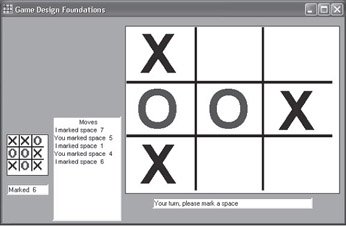` Sub Tic_Tac_Toe() Dim X As Integer Dim MySide As Integer Dim spaceon As Integer Dim FMove As Integer Dim i As Integer Dim value As Integer   X = 0: MySide = -playerMark   If SpaceEmpty(0) Then 'Game still on ' A forced move to win or to block a win is the best mark move     X = Forced_Move(1, MySide)     spaceon = Abs(X) Mod 100     If X <> 0 Then ' A forced move has been calculated       ttt(0, spaceon - 1) = MySide ' either a '1' or a '-1'       Form1.Text1.Text = Form1.Text1.Text + " I marked space " +               Str(spaceon) + " "     End If 'find a space that's not forced     If X = 0 Then ' Initialize the level 1 available mark spaces to an extremely low   value       For i = 0 To 9       Level1Data(i) = -999     Next i ' Call the Min-Max Function where Alpha is very low and Beta is very   high     X = MinMaxValue(1, MySide, -999, 999) ' find the best move ' find the best space to mark from the level 1 list of valid spaces   to mark     spaceon = SpaceEmpty(0) - 1     X = -999     For i = 1 To 9       if Level1Data(i) > X Then         spaceon = i         X= Level1Data(i)       End If     Next i     ttt(0, spaceon - 1) = MySide '  Mark the best space     Form1.Text1.Text = Form1.Text1.Text + " I marked space " +          Str(spaceon) + " "   End If   Form1.Text4.Text = "Marked " + Str(spaceon)   Form1.showSmallBoard ' display current path scenario of board End If Form1.showBigBoard playerDONE = 0 FMove = IsThereaWin(0, playerMark)   If FMove <= -100 Then ' Be prepared to see this message often     Form1.Text1.Text = Form1.Text1.Text + " " + "HURRAY! I WON!"                    + " "     Form1.Text1.Text = Form1.Text1.Text + " Play me again?"     Form1.Text5.Text = "Would you like to play me again?"     playerDONE = 100     Form1.Option2.Visible = True     Exit Sub   End If   If FMove >= 100 Then ' You may never see this message     Form1.Text1.Text = Form1.Text1.Text + " " + "WOW! YOU'VE WON!"                     + "  "     Form1.Text1.Text = Form1.Text1.Text + " Play me again?"     Form1.Text5.Text = "Would you like to play me again?"     playerDONE = 100     Form1.Option2.Visible = True     Exit Sub   End If   If FMove = 0 Then ' No win found and all spaces marked     playerDONE = 0     Form1.Text5.Text = "Your turn, please mark a space"     If SpaceEmpty(0) = 0 Then ' no more empty spaces to mark       Form1.Text1.Text = Form1.Text1.Text + " " + "DRAW!" + " "       Form1.Text1.Text = Form1.Text1.Text + " Play me again?"       Form1.Text5.Text = "Would you like to play me again?"       Form1.Option2.Visible = True       Exit Sub     End If   End If End Sub ' Just like a chess opening database, here are standard ' Tic-Tac-Toe opening marks Sub opening() Dim i As Integer Dim j As Integer Dim k As Integer   Form1.Text5.Text = "" ' clear message ' Check to see if we go first and second   j = 0   For i = 0 To 8     If ttt(0, i) <> 0 Then       j = j + 1       k = i     End If   Next i   If j > 1 Then     Form1.Text5.Text = ""     Tic_Tac_Toe ' find a space to mark     Exit Sub   End If   If j = 0 Then      ' we go first     i = Int(Rnd * 5) ' valid first marks are spaces 1,3,5,7 and 9     i = i * 2  ' we have a random number 0 through 4 so we double it     ttt(0, i) = -playerMark     Form1.Text4.Text = "Marked " + Str(i + 1)     Form1.Text1.Text = Form1.Text1.Text + " I marked space " +                       Str(i + 1) + " "     playerDONE = 0     Form1.showBigBoard     Form1.Text5.Text = "Your turn, please mark a space"     Form1.Picture1.Enabled = True ' Player goes     Exit Sub   End If   If j = 1 Then ' we go second ' valid moves are center and the opposite corner if the opponent ' marked a corner space   i = -1 ' a flag   If k = 4 And i = -1 Then ' center space marked   AAA: i = Int(Rnd * 4)    ' valid first marks are spaces 1,3,7 and 9     If i = 4 Then GoTo AAA ' can't mark the center space     i = i * 2 ' we have a random number 0 through 4 so we double it   End If   If i = -1 Then           ' center space was not marked     i = 4                  ' mark center space (space 5)   End If   ttt(0, i) = -playerMark   Form1.Text4.Text = "Marked " + Str(i + 1)   Form1.Text1.Text = Form1.Text1.Text + " I marked space " + '                    Str(i + 1) + " "   playerDONE = 0   Form1.showBigBoard   Form1.Text5.Text = "Your turn, please mark a space"   Form1.Picture1.Enabled = True ' Player goes   Exit Sub  End If End Sub`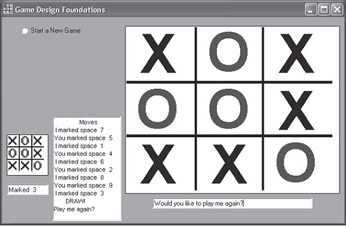` Function MinMaxValue(level As Integer, MySide As Integer, alpha As      Integer, beta As Integer) As Integer Dim WIN       As Integer Dim index     As Integer Dim MTlist    As Long Dim nextspace As Integer Dim X         As Integer Dim succval   As Integer ' reset current level board   Copy_ttt (level)   If SpaceEmpty(level) = 0 Then     MinMaxValue = 0     Exit Function   End If 'find a space that's not forced 'list all open spaces   MTlist = 0 ' List all the open (unmarked) spaces on this level   X = Forced_Move(level, MySide) ' Is there a forced move (a win or a                                  ' block of a win)   If X <> 0 Then                 ' a forced move has been flagged     MTlist = Abs(X Mod 100)      ' space is 1 to 9   Else     For index = 0 To 8       If ttt(level, 8 - index) = 0 Then ' subtract from 8 for            ascending order         MTlist = MTlist * 10 + (9 - index)       End If     Next index   End If   While MTlist > 0     nextspace = MTlist Mod 10          ' next space to mark     MTlist = (MTlist - nextspace) / 10 ' remainder of possible spaces                                        ' to mark     Copy_ttt (level)     ttt(level, nextspace - 1) = MySide     Form1.Text4.Text = "Marked " + Str(nextspace)     Form1.showSmallBoard ' display current path scenario of board     WIN = IsThereaWin(level, MySide)     If WIN >= 100 Then       succval = WIN     Else       succval = -MinMaxValue(level + 1, -MySide, -beta, -alpha)     Endif     If level = 1 Then       ' Save this space's value       Level1Data(nextspace) = succval       If succval = 100 Then ' Winning line found         MinMaxValue = succval         Exit Function       End If     End If 'The Alpha-Beta pruning code     If succval >= beta Then       MinMaxValue = beta       Exit Function     End If     If succval > alpha Then alpha = succval     Wend     MinMaxValue = alpha ' return a draw End Function`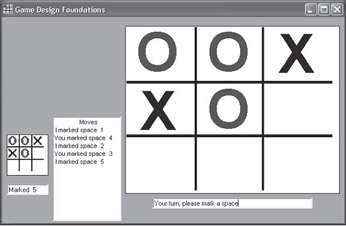`//since C++ start arrays at index Zero we will too int ttt={ {0,0,0,0,0,0,0,0},{0,0,0,0,0,0,0,0},                 {0,0,0,0,0,0,0,0},{0,0,0,0,0,0,0,0},                 {0,0,0,0,0,0,0,0},{0,0,0,0,0,0,0,0},                 {0,0,0,0,0,0,0,0},{0,0,0,0,0,0,0,0}}; int wp={{0,1,2},{3,4,5},{6,7,8}, // Rows               {0,3,6},{1,4,7},{2,5,8}, // Columns               {0,4,8},{2,4,6}};        // Diagonals // Copy a new Tic-Tac-Toe board starting with the previous board void Copy_ttt(int level) {      int index;      if(level<1) return;              // invalid parameter      for(index=0; index<9; index++)           ttt[level][index]= ttt[level-1][index] } // Let's verify if there's an empty space to fill int SpaceEmpty(int level) {      int index;      for(index=0; index<9; index++)           if(ttt[level][index]==0)return(index+1);      return 0;    // no empty space was found } // Let's check to see if there's a forced move to make // A forced move is a winning space or a blocking space to stop  // a loss int Forced_Move(int level, int MySide) {      int index, trys, ttWin;      int OppWin= -3 * MySide;      int MyWin= 3 * MySide;      for(index=0; index<9;index++)           for(trys=0; trys<2; trys++){                if(level>0) Copy_ttt(level);                if(ttt[level][index] == 0){ // empty space                     if(trys ==0)ttt[level][index]= MySide;                     else ttt[level][index]=-MySide; // opponent                ttWin= ttt[level][wp[index] +                     ttt[level][wp[index] +                     ttt[level][wp[index];                if(ttWin == MyWin)return(100 + index); // I win plus                                                    space to move to                else                if(ttWin == OppWin)return(-(100 + index)); // Forced                                                 plus space to block           }      return 0; // No forced move } void Tic_Tac_Toe() { int x=0, MySide= 1, spaceon;      while(SpaceEmpty(0) && x < 100){ // Game still on           x= Forced_Move(1, MySide);           spaceon= abs(x)%100;           if(x != 0)ttt[spaceon]= x/100; // either a '1' or a '-1'           else{          // find a space that's not forced                BestMove(1);    // find the best move           }           x= 0; // reset 'x'      } }`

(This code can be found on the book’s companion CD.)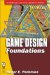Game Design Foundations (Wordware Game and Graphics Library)
ISBN: 1556229739
EAN: 2147483647
Year: 2003
Pages: 179

Similar book on Amazon